Function Repository Resource:

# TableSet

Create a lightweight, named column store of tabular data that is compatible with Query, Select and other SQL-like functions

Contributed by: Edmund B Robinson
 ResourceFunction["TableSet"][names, data] creates a data structure with columns named names and tabular data data.

## Details

Tabular data is the most common data structure seen in data analysis. This form of data does not require much of the functionality needed to manage hierarchical data that is in Dataset. ResourceFunction["TableSet"] fills the gap between accessing a lightweight List with indices and a memory-hungry Dataset with named columns.
ResourceFunction["TableSet"] takes a list of strings for the column names.
ResourceFunction["TableSet"] takes any expression that satisfies MatrixQ for data.
The length of names must equal the number of columns in data.
ResourceFunction["TableSet"][]["prop"] can take the following string properties as "prop":
 "ColumnNames" list of the column names "Data" list of the data "RowCount" number of rows
ResourceFunction["TableSet"] is compatible with the following functions:
 Delete delete a row or multiple rows DeleteDuplicates delete duplicate rows Insert add a row at a specified position JoinAcross join two ResourceFunction["TableSet"] objects by "Left", "Inner" and "Right" specifications Part return parts of the rows and columns Query perform a query using the column names as named slots Select pick out rows according to a criterion TableForm display a ResourceFunction["TableSet"] arranged in an array of rectangular cells Union return a sorted list of all the distinct elements that appear in a ResourceFunction["TableSet"] object
For JoinAcross, "Outer" join and the KeyCollisionFunction option are not implemented.
For Query, use named slots to reference columns.
Since ResourceFunction["TableSet"] is optimised for tabular data, it has a smaller memory footprint than Dataset, which is optimized for hierarchical data. When working with large tabular data, a significant decrease in memory usage can be gained by using ResourceFunction["TableSet"] as compared to Dataset.

## Examples

### Basic Examples (5)

Create a TableSet:

 In:=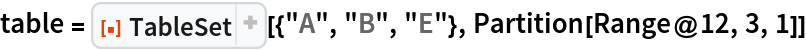Out=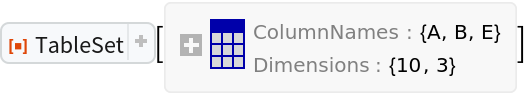View the number of rows:

 In:=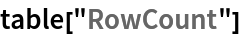Out=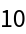Insert a row:

 In:=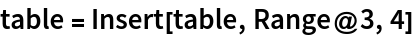Out=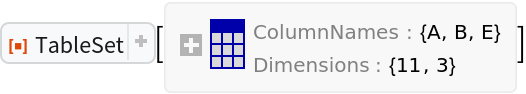View the updated number of rows:

 In:=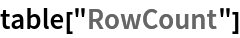Out=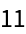View the TableSet in TableForm:

 In:=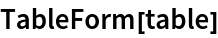Out=### Scope (10)

TableSet has a summary form:

 In:=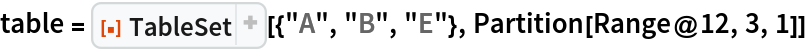Out=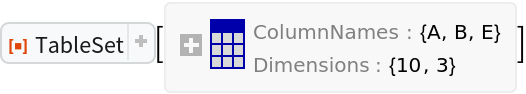Return the column names:

 In:=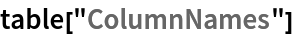Out=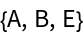Return the row count:

 In:=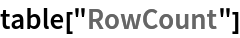Out=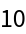Return the data in the TableSet:

 In:=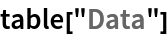Out=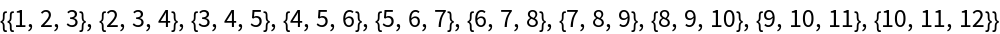Delete a row or rows from a TableSet:

 In:=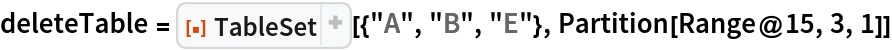Out=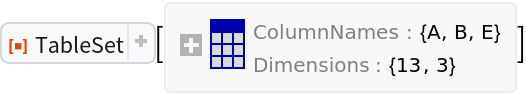TableSet rows before deleting a row:

 In:=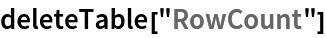Out=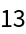Delete row 3:

 In:=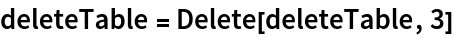Out=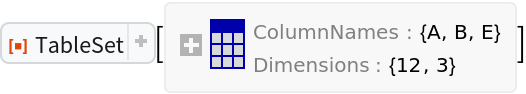Row count after deleting row 3:

 In:=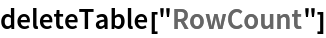Out=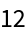Delete row 7 and the last row:

 In:=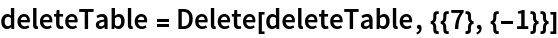Out=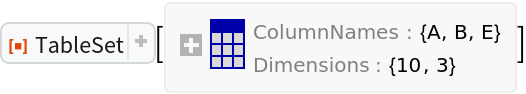Row count after deleting row 7 and the last row:

 In:=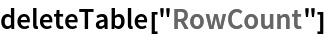Out=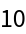Delete duplicate rows:

 In:=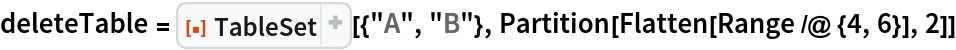Out=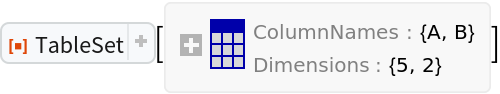The first two data rows are identical to the next two rows:

 In:=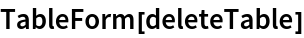Out=Delete duplicate rows:

 In:=Out=Add a row at a specified position:

 In:=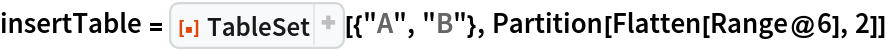Out=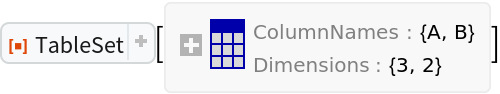Insert a row at position 3:

 In:=Out=Insert rows into position 3 and the last position:

 In:=Out=For JoinAcross, "Outer" and KeyCollisionFunction are not implemented:

 In:=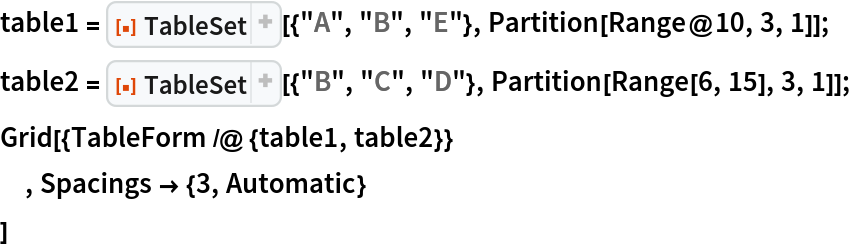Out=Evaluate "Left", "Inner" and "Right" joins:

 In:=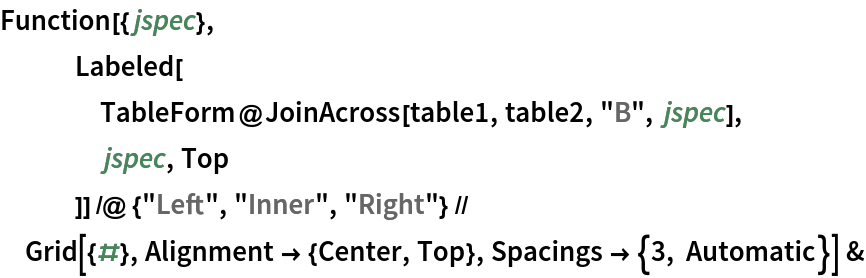Out=Return parts of the rows and columns:

 In:=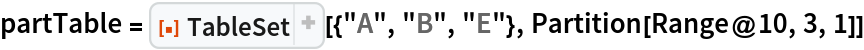Out=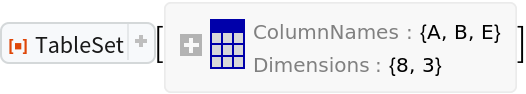Return the first two rows:

 In:=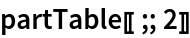Out=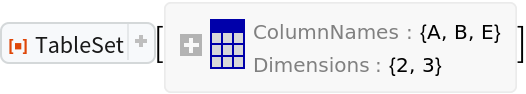Return every other row:

 In:=Out=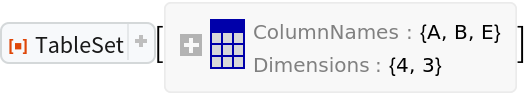Return the last column:

 In:=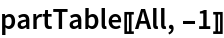Out=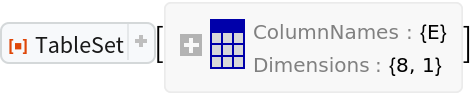Use named slots to reference TableSet columns in Query:

 In:=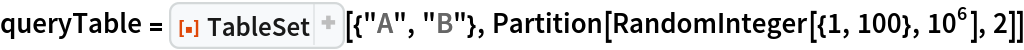Out=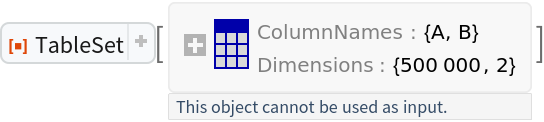Query a Histogram of the sum of the columns:

 In:=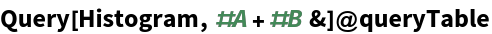Out=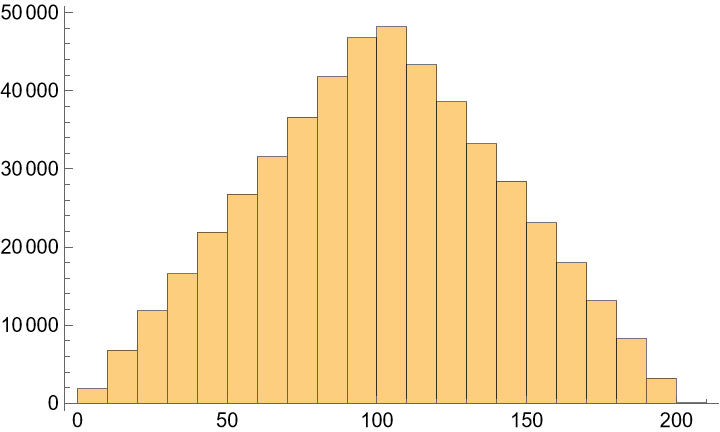Add columns based on calculations on existing columns:

 In:=Out=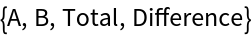Pick out rows according to a criterion:

 In:=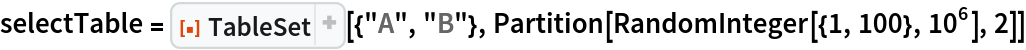Out=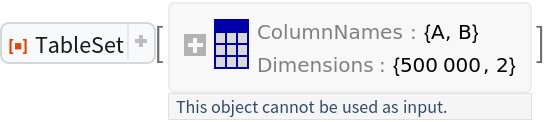Select rows with column A > 4 and B < 12:

 In:=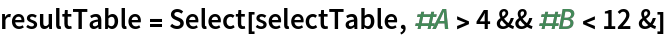Out=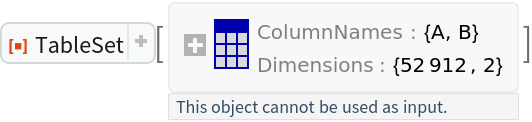Compare the row count of the original and result table:

 In:=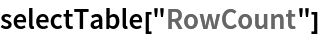Out=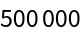In:=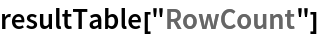Out=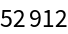Display the TableSet arranged in an array of rectangular cells:

 In:=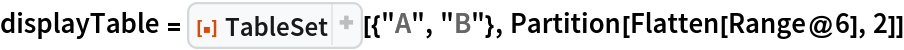Out=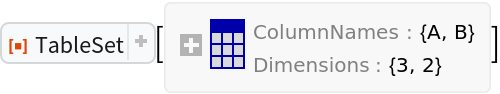In:=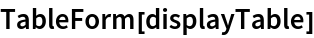Out=Create TableSets with example data:

 In:=View TableSetobjects in TableForm:

 In:=Out=Union gives the union of the rows maintaining the column names from the first table:

 In:=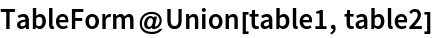Out=### Properties and Relations (4)

When working with large tabular data, a significant decrease in memory usage can be gained by using TableSet as compared to Dataset. For example, with a two-column table of integer values, the ByteCount difference quickly widens with the record count as the graph tooltips and the following table demonstrate:

 In:=Create the data:

 In:=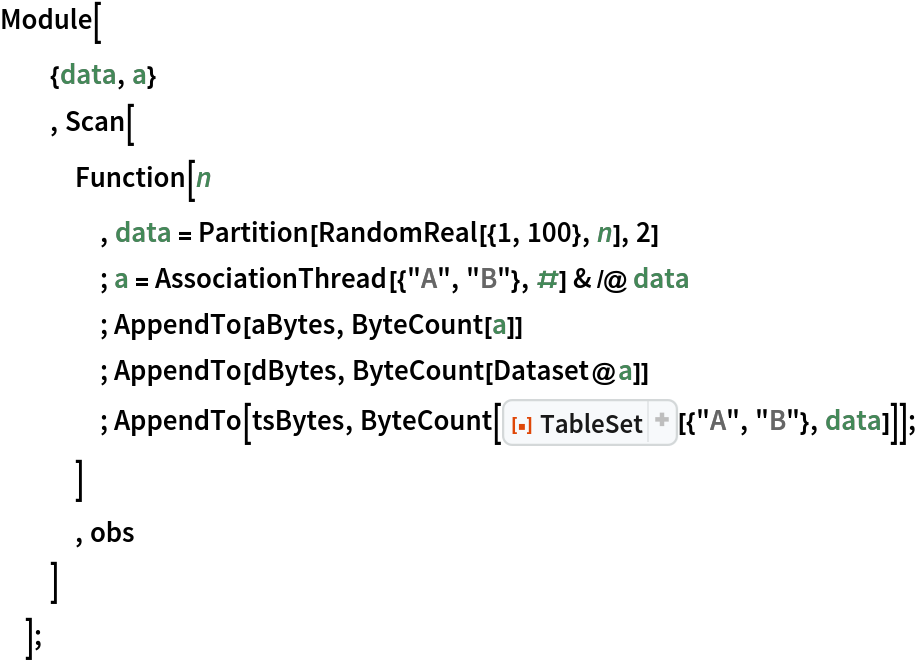Plot it:

 In:=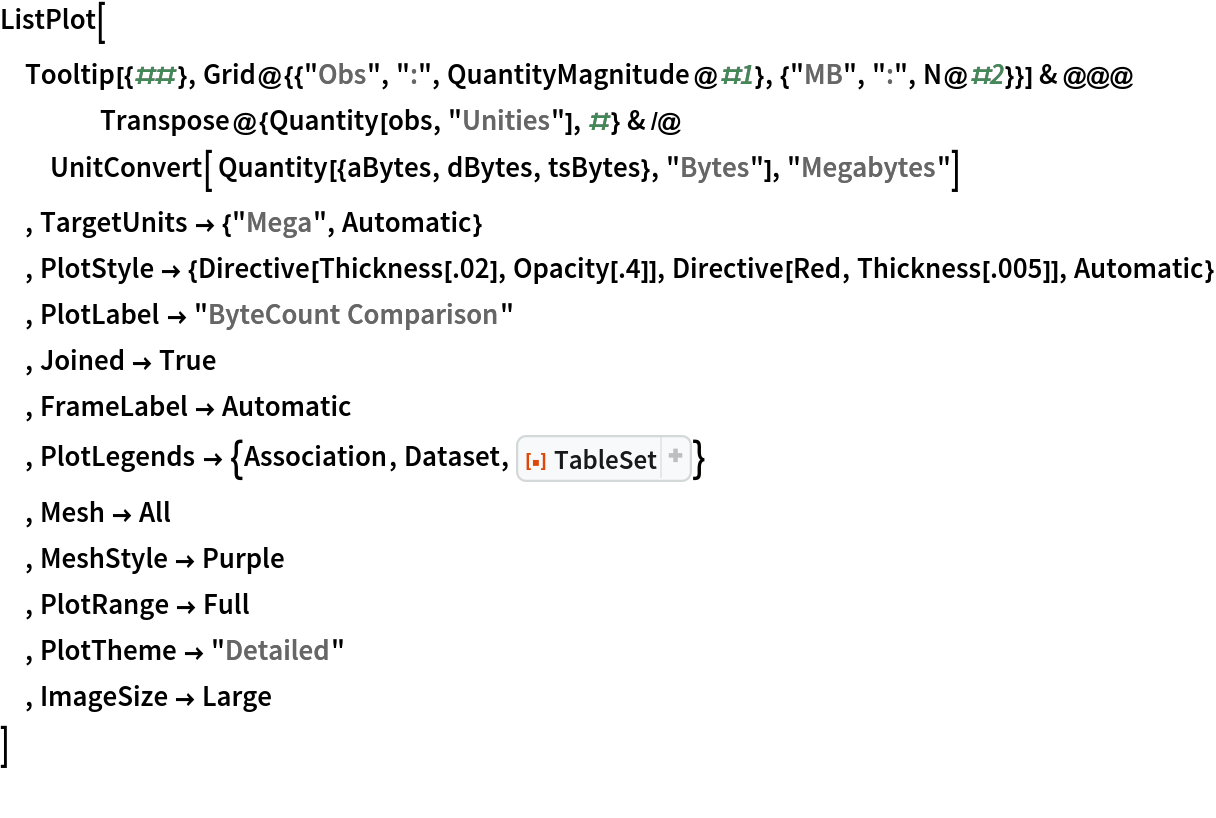Out=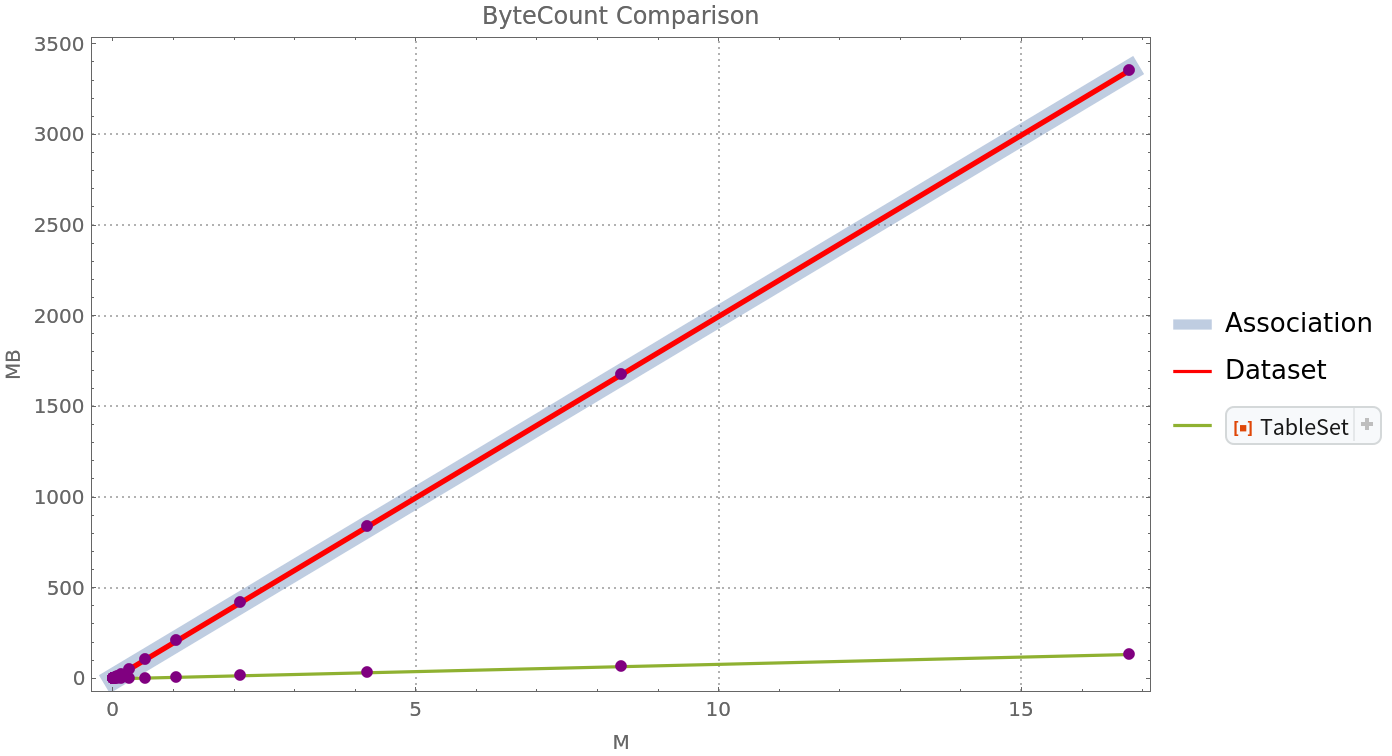See the data in a table:

 In:=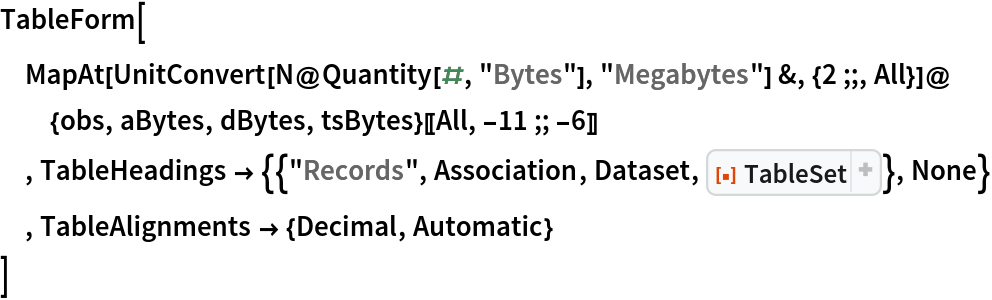Out=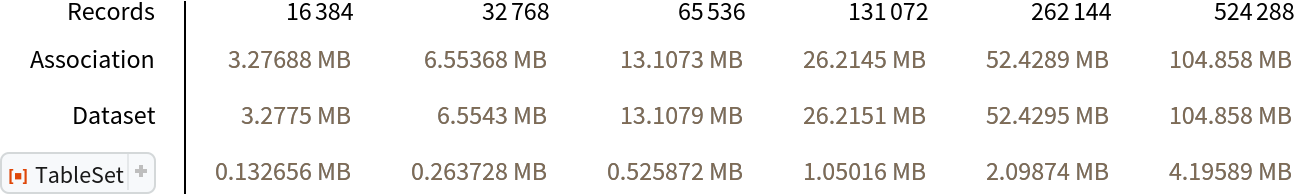### Possible Issues (2)

A Query to add columns returns a TableSet:

 In:=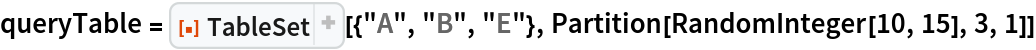Out=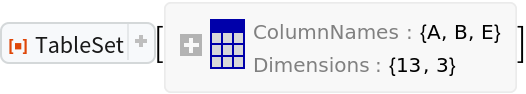In:=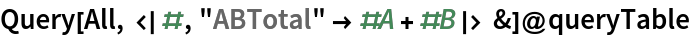Out=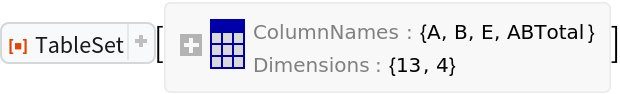However, a general query does not return a TableSet:

 In:=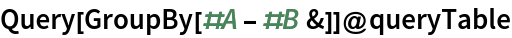Out=## Publisher

Edmund B Robinson

## Version History

• 1.0.0 – 10 August 2021